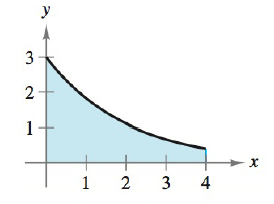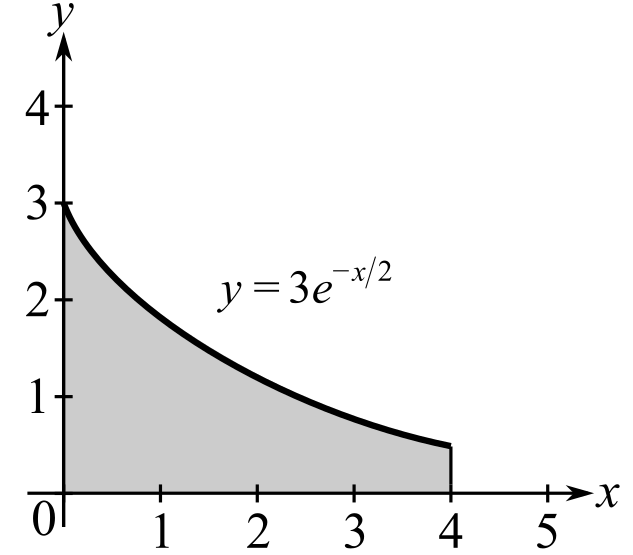Chapter 5.4, Problem 13E### Calculus: An Applied Approach (Min...

10th Edition
Ron Larson
ISBN: 9781305860919

#### Solutions

Chapter
Section### Calculus: An Applied Approach (Min...

10th Edition
Ron Larson
ISBN: 9781305860919
Textbook Problem
12 views

# Finding Area by the Fundamental Theorem In Exercises 9-16, find the area of the region. See Example 2. y = 3 e − x / 2To determine

To calculate: The area of the region y=3ex/2 in the provided figure.Explanation

Given Information:

The function is y=3ex/2 and the provided figure is:

Formula used:

The fundamental theorem of calculus states that,

If f is integrable on interval [a,b] then abf(x)dx=F(b)F(a).

The integration formula is exdx=ex+C.

Calculation:

Consider the figure.

To find the area of the region, fundamental theorem of calculus can be used.

Integrate the function from x=0 to x=0 as shown below,

043ex/2dx

Multiply and divide by (2),

043ex/2dx=043ex/2(2)dx(2)

Now find antiderivative by applying the integration formula,

### Still sussing out bartleby?

Check out a sample textbook solution.

See a sample solution

#### The Solution to Your Study Problems

Bartleby provides explanations to thousands of textbook problems written by our experts, many with advanced degrees!

Get Started

#### What is the value of (X + 1) for the following scores: 0, 1, 4, 2? a. 8 b. 9 c. 11 d. 16

Essentials of Statistics for The Behavioral Sciences (MindTap Course List)

#### In Exercises 1520, simplify the expression. 18. (2x3) (3x2) (16x1/2)

Applied Calculus for the Managerial, Life, and Social Sciences: A Brief Approach

#### The third partial sum of is:

Study Guide for Stewart's Multivariable Calculus, 8th

#### Use a Table of Integrals to evaluate .

Study Guide for Stewart's Single Variable Calculus: Early Transcendentals, 8th